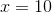Trigonometry : Proportions in Similar Triangles

Example Questions

Example Question #1 : Proportions In Similar Triangles

Which of the following shifts are incorrect?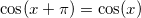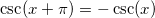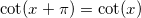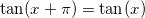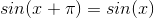Explanation:

The actual shift for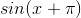is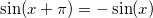.

Example Question #1 : Similar Triangles

A right triangle has side lengths of,, and. A similar right triangle has sides of,, and. What isThere is not enough information to determine.Explanation:

Similar triangles by defnition have proportional sides. We can divide corresponding parts in this case to find the scale factor.

Corresponding parts are the two smallest sides, the medium sides, and the largest sides.

Thus: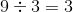is the scale factor.

Then, we use this to find the missing side.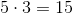Therefore,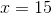.

Example Question #32 : Triangles

Corresponding sides of two triangles have measures ofand. If another side of the small triangle is, what is the value of the bigger triangles corresponding side?Explanation:

We can find the scale factor by dividing 18 by 6.

We get that the scale factor is 3.

Multiplying the other side by 3, we get the new side, 21.

Example Question #11 : Similar Triangles

Which set of the following triangle dimensions does NOT have the same proportions as a 3-4-5 triangle?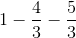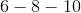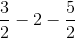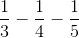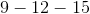Explanation:

In order to determine whether if the dimensions of the triangle are of the same proportions, the ratios of the dimensions must also be the same as the 3-4-5 triangle.

The following scale factors multiplied to the 3-4-5 triangle yield similar proportions.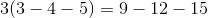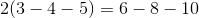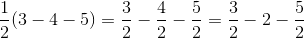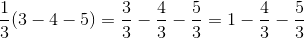The only dimensions that cannot be attained by multiplying a particular scale factor with the 3-4-5 is:Example Question #12 : Similar Triangles

These triangles are similar. Use this to solve for x: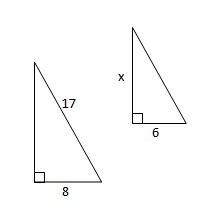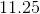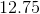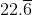Explanation:

Initially we are given the hypotenuse and one leg of the large triangle, but both legs of the small triangle. To set up a proportion, we need to know both legs of the large triangle, and we can solve for the missing one using the pythagorean theorem:, where a and b are the legs and c is the hypotenuse.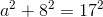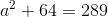subtract 64 from both sides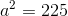take the square root of both sides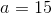Now that we have both legs, we can see that 15 corresponds with x, and 8 corresponds with 6, so we can set up a proportion to solve for x: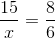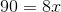divide both sides by 8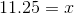Example Question #13 : Similar Triangles

These two triangles are similar. Solve for x: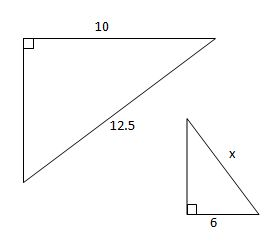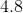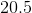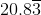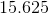Explanation:

Before we can set up a proportion, we need to know the third side of the larger triangle, since that side is actually the one that corresponds to 6, since both are the shortest sides in their respecitve triangles. We can solve for that side using the Pythagorean Theorem,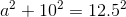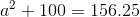subtract 100 from both sides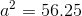take the square root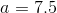Now we can set up a proportion comparing corresponding sides to solve for x: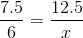cross-multiply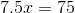divide by 7.5